# 3.深度强化学习——PPO(Proximal Policy Optimization)算法资料+原理整理

## 一. PPO资料

PPO原理讲解BLOG①：这篇blog详细讲了PPO算法的产生过程及原理，包含部分理论推导，看完这篇blog基本就理解PPO了。
PPO原理讲解BLOG②：可参考，其中包含部分tensorflow实现代码。

## 二. PPO原理简述

### 2.1 背景

L ( θ ) = E [ l o g π ( a ∣ s , θ ) f ( s , a ) ] = ∑ l o g π ( a ∣ s , θ ) f ( s , a ) L(\theta) = \mathrm{E} [log\pi(a|s,\theta)f(s,a)]= \sum log\pi(a|s,\theta)f(s,a)L (θ)=E [l o g π(a ∣s ,θ)f (s ,a )]=∑l o g π(a ∣s ,θ)f (s ,a ) L P G ( θ ) = E ^ t [ log ⁡ π θ ( a t ∣ s t ) A ^ t ] L^{P G}(\theta)=\hat{\mathbb{E}}{t}\left[\log \pi{\theta}\left(a_{t} \mid s_{t}\right) \hat{A}_{t}\right]L P G (θ)=E ^t ​[lo g πθ​(a t ​∣s t ​)A ^t ​]

T D ( λ ) TD(\lambda)T D (λ)可以理解为对n个n-step 的估计值作加权平均得到的结果

### 2.2 原理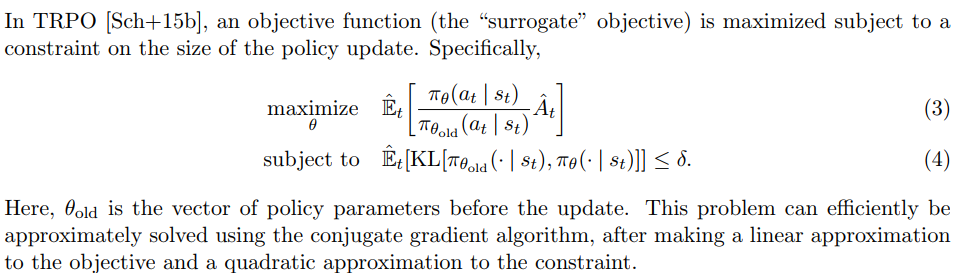IS实际上实现的过程就是用一种分布的采样均值来代替另一种分布的期望

PPO的主要贡献是提出了clipped surrogate objective，如下所示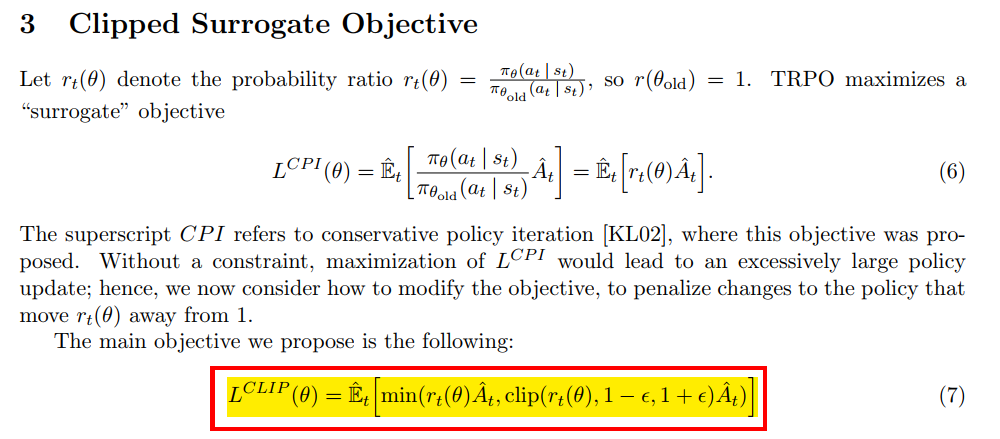note that the probability ratio r r r is clipped at 1 − ϵ 1-\epsilon 1 −ϵor 1 + ϵ 1+\epsilon 1 +ϵdepending on whether the advantage is positive or negative

ϵ \epsilon ϵ通常来说是一个比较小的值，论文中作者设置为0.2时在比较好的实验效果，这个clip操作实际上是限制了策略更新的幅度，避免策略的一次更新偏离太远。

PPO论文中还给出了对TRPO方法的一种近似/替代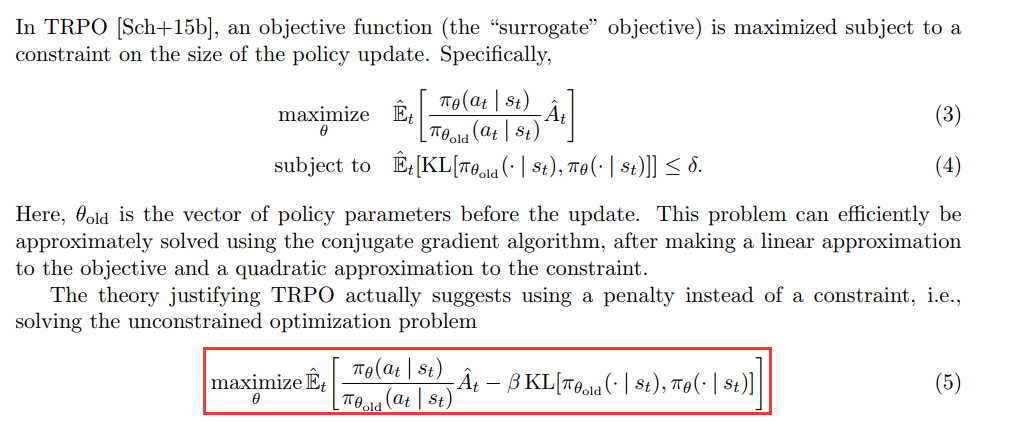PPO最终的loss如下：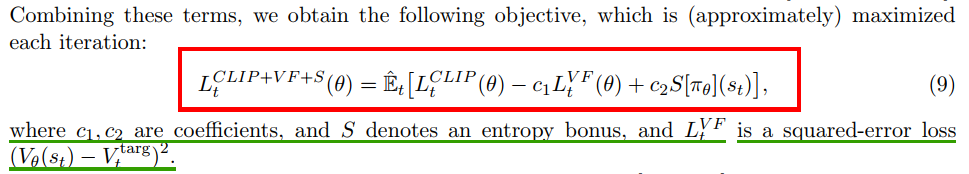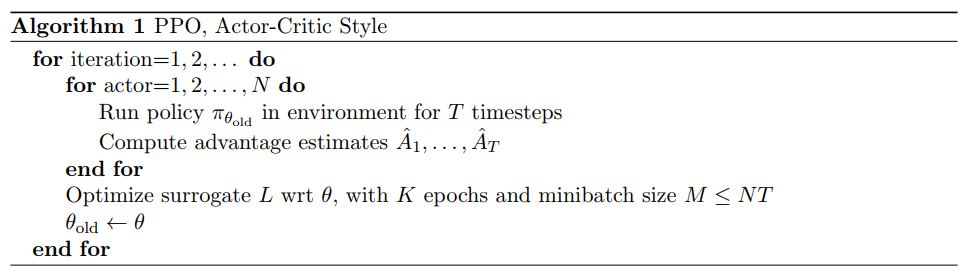openai dota five对sample reuse有不同的定义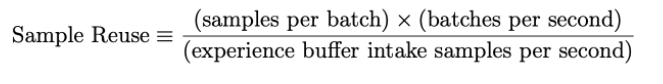Original: https://blog.csdn.net/qq_38587510/article/details/123463954
Author: EdenGabriel
Title: 3.深度强化学习------PPO(Proximal Policy Optimization)算法资料+原理整理

## 用Typescript 的方式封装Vue3的表单绑定，支持防抖等功能。

Vue3 的父子组件传值、绑定表单数据、UI库的二次封装、防抖等，想来大家都很熟悉了，本篇介绍一种使用 Typescript 的方式进行统一的封装的方法。 Vue3对于表单的绑定提供了一种简单的方式：...